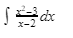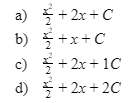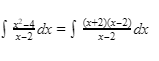Integral Equation homework Help at TutorEye

# Best Homework Help For Integral Equation

## Top Questions

t schedule 3 people for the day my % scheduled is 78.57% My question is how did the computer come up with the 78.57%?
View More

I would love to get a written solution. I did the other proof tree that you can see in the attaached files. So its supposed to look like that Thank You.
View More

xtbook. i really need in in half an hour
View More

## Integral Equation:

Integral Equation is the equation with unknown function inside the one or more  integral signs. It carries a vast application in framing mathematical models for several real life engineering problems. It contributes in the field of quantum mechanics, waves and theoretical physics.

## Integral Equation Sample Questions:

Question 1: Find lambda :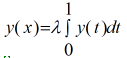(a) 0

(b) 1

(c) 2

(d) 3

Explanation: Let y(x) = c

Question 2: Solve: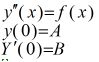(a)(b)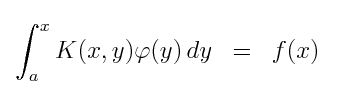(c)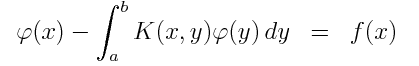(d) None of the above

Explanation: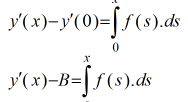Question 3: Solve :(a)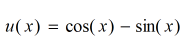(b)  ∫ a^x dx = (ax/ln a) + C ; a>0,  a≠1

(c) (1/x) dx = ln |x| + C

(d) ∫ csc x ( cot x) dx = – csc x + C

Explanation: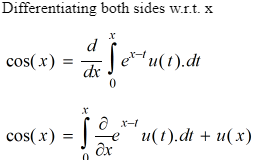Question 4: Find lambda :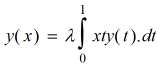if y(x)=x

(a) 1

(b) 2

(c) 3

(d) 4

Explanation: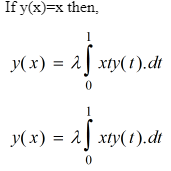Question 5: Solve:(a)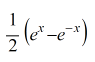(b)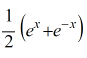(c)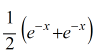(d)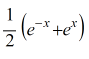Explanation: In this problem f(x)=x and k(x-y)=x-y
Take the laplace transform on both sides.

Question 6: For the integral equation: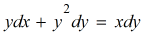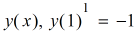then y(-3) is

(a) -2,1

(b) -3,1

(c) 1,1

(d) 1,-1

Explanation: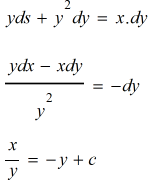Question 7: Find the eigenvalue lambda  :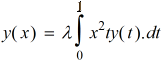(a) 1/2

(b) 1/4

(c) 1/3

(d) 1/6

Explanation: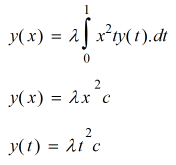Question 8: Solve: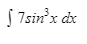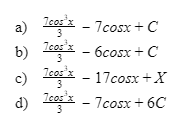Explanation: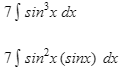Question 9: An object falling from a bridge with velocity vt=-5t-4 ms. Find the height of the bridge, if the object touches the ground after t=10 seconds.

(a) 290 m

(b) 90 m

(c) 200 m

(d) 120 m

Explanation: To find the vertical position of the object, we need to integrate the velocity equation with respect to time.

Question 10: Solve: